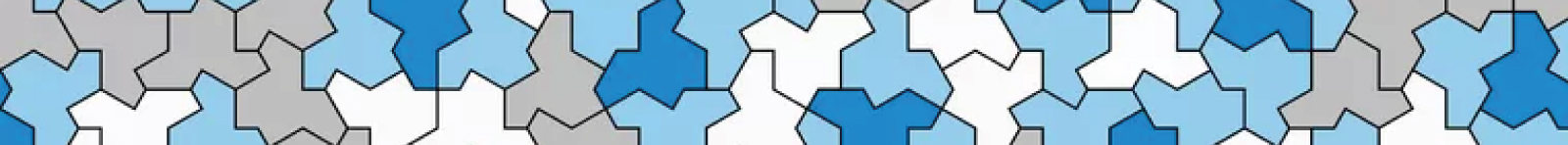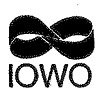# Lerarenopleidingen Science en Wiskunde/Rekenen# HewetHerverkaveling eindexamenprogramma’s wiskunde I en II vwo Project 1981-1985 Uitlijning van Wiskunde A (en B).

### Proefschrift Jan de Lange

Engelstalige abstract

The Mathematics A curriculum was developed between 1981 and 1985 as the main result of the Hewet project. The first chapter describes the events leading to this project, taking as a starting point the Royaumont conference in 1959. Furthermore the basic scheme of this project is given, which seems interesting from the point of view of innovation. The second chapter deals with contents, methods and form of Mathematics A, and gives a sketch of a theoretical framework for instruction as well. To introduce the reader as soon as possible to the proper atmosphere the content description has been illustrated with examples from the experimental student material. After the content-description an analysis of Mathematics A is given. That means: an analysis not only based on the experimental material, but also on reflection on years of classroom observations, discussions with students, teachers and teacher trainers. This means an evaluation from the inside: the analysis is carried out by one of the developers of the Mathematics A curriculum. One of the goals of Mathematics A that is very important and present at all times is the ability to mathematize.

A general discussion about Mathematization is followed by a more specific one: Mathernatization in Mathematics A. Two ways of mathematization are being distinguished:
# Mathematization as part of the problem solving process, which we might call: Applied Mathematization.
# Mathematization as a way to introduce mathematical concepts, which will be referred to as Conceptual Mathematization.
The latter has a strong three-dimensional goal aspect: it is a didactical component of the learning process that contributes to a clarification of the process and product goals. (One dimension goal: general goals, two dimensions goal: behaviour and content component.) [I] On mathematization we finally discuss some aspects of a theoretical framework for Mathematics A instruction. A major role in the experimental student material is played by the context. After mathematization this is the second point for discussion in our analysis of Mathematics A. The third and last point that will be treated in this chapter is clearly a onedimensional goal: how to create a critical attitude? Examples of mathematical material and work of students and teachers enable the author to support his views and the reader to acquaint himself with the kinds of experience of mathematics education in upper secondary school that were propagated by the team that carried out the project. The way how students and teachers perceive Mathematics A will be discussed; comparing their views with our description gives remarkable results.

Mathematics A is clearly a curriculum and methodology that fits in directly with Realistic Mathematics Education. The shift from Mechanistic-Structuralistic Mathematics Education to Realistic Mathematics Education that takes place in the Netherlands is described and followed by an example that shows the sharp distinction between the two philosophies. Emphasis on applications in Mathematics A makes it worthwhile to spend time on the continuing discussion about the dichotomy between pure and applied mathematics.

This is taken care of in part 4 where we also discuss the sharp break-away from digital or analytical mathematics towards analog mathematics. Maybe another false dichotomy? The third chapter gives the result of developmental research carried out among students and teachers of the experimenting schools. Not only is a chapter on experiences a necessity when describing a project like the Hewet project but it also gives the opportunity for two interesting observations that are strongly related to other parts of this study. A general observation is that most teachers that took part in the experiments experience the new Mathematics A curriculum as positive. And there is a noteworthy positive shift in their attitude. Both students and teachers gave in their comments considerable support for our views on Mathematics A as expressed in the previous chapter.
Finally teachers made clear that one of the problems with the Mathematics A curriculum lies in its achievement testing. The problems mentioned and experiences by teachers when preparing tests for Mathematics A lead to the next phase of our study. In the fourth chapter we describe how teacher-made tests were collected and analyzed. This lead to the conclusion that timed tests were not operationalizing the goals of Mathematics A in a satisfactory way.

The analysis of Mathematics A, the results of experiences by teachers and students and the outcome of the research on the timed-tests all converge to the same conclusion: an exploratory study of the possibilities of achievement testing in an alternative way was needed. This study is described in next chapter. In the fifth chapter the principles behind these tasks are first stated and discussed. These principles are followed by a description of four kinds of alternative tasks: